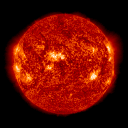Current Australian State Warnings WebcamCurrent Tasmanian Fire Incidents

### MoonSunrise: 07:30
Sunset: 17:08
Daylight: 09:38

Current

Earth
showing
Cloud Cover
Moonrise: 07:08 (28/07/2014)
Moonset: 17:37 (28/07/2014)
New moon
1% Illuminated

 First Quarter Moon Full Moon Last Quarter Moon New Moon04/08/2014 10:50 04 August 2014 00:50 GMT 11/08/2014 04:09 10 August 2014 18:09 GMT 17/08/2014 22:26 17 August 2014 12:26 GMT 26/08/2014 00:13 25 August 2014 14:13 GMT

 Vernal Equinox Start of Spring Summer Solstice Start of Summer Autumn Equinox Start of Fall Winter Solstice Start of Winter23/09/2014 12:29 23 September 2014 02:29 GMT 22/12/2014 10:03 21 December 2014 23:03 GMT 21/03/2014 03:57 20 March 2014 16:57 GMT 21/06/2014 20:51 21 June 2014 10:51 GMT

 SUN DATASun Position Chart at time of page visitDay / Twilight / Night 24-hour "clock" at time of page visitClock LegendNoon is technically when the Sun is at Zenith. Morning civil twilight is when the Sun is 6� below the horizon and ends at Sunrise. Evening civil twilight begins at Sunset and ends when the Sun reaches 6� below the horizon. Nautical twilight is when the Sun is between 6� and 12� below the horizon. Astronomical twilight is when the Sun is between 12� and 18� below the horizon. Sun Functions CURRENT TIME00:17CURRENT EVENTNight TimeTODAYS EVENTS05:48 - Astronomical Twilight starts06:22 - Nautical Twilight starts06:56 - Civil Twilight starts07:29 - SUNRISETwilight hours17:07 - SUNSET17:40 - Civil Twilight ends18:14 - Nautical Twilight ends18:48 - Astronomical Twilight ends

MOON DATA
Moon Phases Calemdar updated at time of page visit

Phases of the Moon for current month

# //JavaScript modification T. Dacre 1999 //based on a BASIC program MOON EFFECTS by Bradley E. Schaefer // This program helps anyone who needs to know the Moon's // phase (age) on // any date within several thousand years in the past or future. // To illustrate its application, Bradley Schaefer applied it // to a number of famous events influenced by the Moon in // World War II. His article appeared in Sky & Telescope for // April 1994, page 86. mydate = new Date(); daytoday=mydate.getDate(); calc(mydate.getMonth(),mydate.getFullYear()); function MP(day){ day2=new Date(); day2=day; Date.UTC(day); var Y=day2.getFullYear(); var M=day2.getMonth()+1; var D=day2.getDate(); var YY,MM,K1,K2,K3,J,V,IP,AG; YY=Y-Math.floor((12-M)/10); MM=M+9; if (MM>=12) {MM=MM-12}; K1=Math.floor(365.25*(YY+4712)); K2=Math.floor(30.6*MM+.5); K3=Math.floor(Math.floor((YY/100)+49)*.75)-38; J=K1+K2+D+59; if (J>2299160) {J=J-K3}; V=(J-2451550.1)/29.530588853; V=V-Math.floor(V); if (V<0) {V=V+1}; IP=V; AG=IP*29.53; IP=IP*Math.PI*2 return IP; } function calceclp(calcmonth,calcyear){ //Eclipse's EC1=0; EC2=0; EM1=""; EM2=""; R1=3.14159265/180; U=0; Y=calcyear; calcmonth++; G=1; K0=Math.floor((Y-1900)*12.3685); T=(Y-1899.5)/100; T2=T*T; T3=T*T*T; J0=2415020+29*K0; F0=0.0001178*T2-0.000000155*T3; F0=F0+0.75933+0.53058868*K0; F0=F0-0.000837*T-0.000335*T2; J0=J0+Math.floor(F0); F0=F0-Math.floor(F0); M0=K0*0.08084821133; M0=360*(M0-Math.floor(M0))+359.2242; M0=M0-0.0000333*T2; M0=M0-0.00000347*T3; M1=K0*0.07171366128; M1=360*(M1-Math.floor(M1))+306.0253; M1=M1+0.0107306*T2; M1=M1+0.00001236*T3; B1=K0*0.08519585128; B1=360*(B1-Math.floor(B1))+21.2964; B1=B1-0.0016528*T2; B1=B1-0.00000239*T3; for (K9=1;K9<=27;K9=K9+2){ J=J0+14*K9; F=F0+0.765294*K9; K=K9/2; M5=(M0+K*29.10535608)*R1; M6=(M1+K*385.81691806)*R1; B6=(B1+K*390.67050646)*R1; F=F-0.4068*Math.sin(M6); F=F+(0.1734-0.000393*T)*Math.sin(M5); F=F+0.0161*Math.sin(2*M6); F=F-0.0104*Math.sin(2*B6); F=F-0.0074*Math.sin(M5-M6); F=F-0.0051*Math.sin(M5+M6); F=F+0.0021*Math.sin(2*M5); F=F+0.5/1440; J=J+Math.floor(F); F=F-Math.floor(F); //100 REM LUNAR ECLIPSE SUBROUTINE function eclipser(){ estr=""; D7=0; if (Math.abs(Math.sin(B6))>0.36){return 0} S=5.19595-0.0048*Math.cos(M5); S=S+0.0020*Math.cos(2*M5); S=S-0.3283*Math.cos(M6); S=S-0.0060*Math.cos(M5+M6); S=S+0.0041*Math.cos(M5-M6); C1=0.2070*Math.sin(M5); C1=C1+0.0024*Math.sin(2*M5); C1=C1-0.0390*Math.sin(M6); C1=C1+0.0115*Math.sin(2*M6); C1=C1-0.0073*Math.sin(M5+M6); C1=C1-0.0067*Math.sin(M5-M6); C1=C1+0.0117*Math.sin(2*B6); D9=Math.abs(S*Math.sin(B6)+C1*Math.cos(B6)); U=0.0059+0.0046*Math.cos(M5); U=U-0.0182*Math.cos(M6); U=U+0.0004*Math.cos(2*M6); U=U-0.0005*Math.cos(M5+M6); RP=1.2847+U; RU=0.7404-U; MPE=(1.5572+U-D9)/0.545; if (MPE<0){return} MU=(1.0129-U-D9)/0.545; D5=1.5572+U; D6=1.0129-U; D7=0.4679-U; N=(0.5458+0.04*Math.cos(M6))/60; D5=((D5*D5-D9*D9)*(D5*D5-D9*D9))/N; if (!(MU<=0)){ D6=((D6*D6-D9*D9)*(D6*D6-D9*D9))/N}; if (!(MU<=1)){ D7=((D7*D7-D9*D9)*(D7*D7-D9*D9))/N}; Jq=J; Fq=F; G=1; if ( Y<1583){G=0} Fq=Fq+0.5; if (!(Fq<1)){ Fq=Fq-1; Jq=Jq+1; } if (G==1){ A1=Math.floor((Jq/36524.25)-51.12264); A=Jq+1+A1-Math.floor(A1/4) } else {A=Jq}; B=A+1524; C=Math.floor((B/365.25)-0.3343); Dq=Math.floor(365.25*C); E=Math.floor((B-Dq)/30.61); Dq=B-Dq-Math.floor(30.61*E)+Fq; Mq=E-1; Yq=C-4716; if (E>13.5){Mq=Mq-12}; if (Mq<2.5) {Yq=Yq+1}; D1=Math.floor(Dq); H=24*(Dq-D1); H1=Math.floor(H); M9=Math.floor(60*(H-H1)); if (M9<10){M9="0"+M9}; if (MU<0 ){estr=estr+"Partial Eclipse, "} else{ D6=Math.floor(D6+0.5); estr=estr+"Total Eclipse - "; } if (!(MU<0 )){ D6=Math.floor(D6+0.5); D7=Math.floor((D7+0.5)*10)/10; estr=estr+D7+"min duration, "; } estr=estr+"Maximum phase "+H1+":"+M9+"UT"; if ((Mq == calcmonth) && (Yq == calcyear)) { if (EC1==0) {EC1=D1;EM1=estr} else {EC2=D1;EM2=estr} } } } } function calc(calcmonth,calcyear){ calceclp(calcmonth,calcyear); //10 REM NEW AND FULL MOONS //12 REM //14 REM var Y,JJ,K1,K2,K3,D,M,MM,FM1,FM2,NM1,NM2; FM1=0; FM2=0; NM1=0; NM2=0; Tt1=""; Tt2=""; Tt3=""; Tt4=""; Y=calcyear; // year M=calcmonth; M++; D=-1; YY=Y-Math.floor((12-M)/10); MM=M+9; if(MM>=12){MM=MM-12} K1=Math.floor(365.25*(YY+4712)); K2=Math.floor(30.6*MM+.5); K3=Math.floor(Math.floor((YY/100)+49)*.75)-38; JJ=K1+K2+D+59; if (JJ>2299160){JJ=JJ-K3} var A,B,C,Dq,E,G,D1,H1,M9,Mq,H,Yq,A1,Fq,Jq; var R1,U,K0,T,T2,T3,J0,F0,J,M0,M1,B1,M5,M6,K9,F; R1=3.14159265/180; U=0; K0=Math.floor((Y-1900)*12.3685); T=(Y-1899.5)/100; T2=T*T; T3=T*T*T; J0=2415020+29*K0; F0=0.0001178*T2-0.000000155*T3; F0=F0+0.75933+0.53058868*K0; F0=F0-0.000837*T-0.000335*T2; J=J+Math.floor(F); F=F-Math.floor(F); M0=K0*0.08084821133; M0=360*(M0-Math.floor(M0))+359.2242; M0=M0-0.0000333*T2; M0=M0-0.00000347*T3; M1=K0*0.07171366128; M1=360*(M1-Math.floor(M1))+306.0253; M1=M1+0.0107306*T2; M1=M1+0.00001236*T3; B1=K0*0.08519585128; B1=360*(B1-Math.floor(B1))+21.2964; B1=B1-0.0016528*T2; B1=B1-0.00000239*T3; for(K9=0;K9<29;K9++){ J=J0+14*K9; F=F0+0.765294*K9; K=K9/2; M5=(M0+K*29.10535608)*R1; M6=(M1+K*385.81691806)*R1; B6=(B1+K*390.67050646)*R1; F=F-0.4068*Math.sin(M6); F=F+(0.1734-0.000393*T)*Math.sin(M5); F=F+0.0161*Math.sin(2*M6); F=F+0.0104*Math.sin(2*B6); F=F-0.0074*Math.sin(M5-M6); F=F-0.0051*Math.sin(M5+M6); F=F+0.0021*Math.sin(2*M5); F=F+0.0010*Math.sin(2*B6-M6); J=J+Math.floor(F); F=F-Math.floor(F); Jq=J; Fq=F; G=1; if ( Y<1583){G=0} Fq=Fq+0.5; if (!(Fq<1)){ Fq=Fq-1; Jq=Jq+1; } if (G==1){ A1=Math.floor((Jq/36524.25)-51.12264); A=Jq+1+A1-Math.floor(A1/4) } else {A=Jq}; B=A+1524; C=Math.floor((B/365.25)-0.3343); Dq=Math.floor(365.25*C); E=Math.floor((B-Dq)/30.61); Dq=B-Dq-Math.floor(30.61*E)+Fq; Mq=E-1; Yq=C-4716; if (E>13.5){Mq=Mq-12}; if (Mq<2.5) {Yq=Yq+1}; D1=Math.floor(Dq); H=24*(Dq-D1); H1=Math.floor(H); M9=Math.floor(60*(H-H1)); if (M9<10){M9="0"+M9}; if ((U==0) && (Mq==M) && (Yq==Y)){if (NM1==0){NM1=D1;Tt1=H1+":"+M9} else {if(NM2==0){NM2=D1;Tt2=H1+":"+M9}}}; if ((U==1) && (Mq==M) && (Yq==Y)){if (FM1==0){FM1=D1;Tt3=H1+":"+M9} else {if(FM2==0){FM2=D1;Tt4=H1+":"+M9}}}; U=U+1; if (U==2){U=0}; } var temp,dayoffset,days,i,j,monthnames,todayday; today=new Date(Date.UTC(calcyear,calcmonth,10,1,1)); month=new Date(Date.UTC(calcyear,calcmonth,10,1,1)); todayday=today.getDate(); month.setDate(1); dayoffset=month.getDay()-1; if (dayoffset==-1){dayoffset=6}; today.setDate(1); today.setMonth(today.getMonth()+1); days=(today-month)/1000/60/60/24; month.setHours(11); monthnames=["January","February","March","April","May","June","July","August","September","October","November","December"]; document.write('<H2 align="center"><font color="#FFFFF9">Phases of the Moon ', monthnames[month.getMonth()]," ",month.getFullYear(),"</font></2>"); document.write('<table border="0" align="center" > <tr bgcolor="#9999FF"> <td width="65"> <div align="center"><b><font color="#FFFFFF">Mon</font></b></div> </td> <td width="65"> <div align="center"><b><font color="#FFFFFF">Tue</font></b></div> </td> <td width="65"> <div align="center"><b><font color="#FFFFFF">Wed</font></b></div> </td> <td width="65"> <div align="center"><b><font color="#FFFFFF">Thur</font></b></div> </td> <td width="65"> <div align="center"><b><font color="#FFFFFF">Fri</font></b></div></td> <td width="65"> <div align="center"><b><font color="#FFFFFF">Sat</font></b></div> </td> <td width="65"> <div align="center"><b><font color="#FFFFFF">Sun</font></b></div> </td> </tr>'); MD1="New Moon "+Tt1; MD2="New Moon "+Tt2; MD3="Full Moon "+Tt3; MD4="Full Moon "+Tt4; if (FM1==EC1){MD3=MD3+String.fromCharCode(10)+EM1} if (FM2==EC1){MD4=MD4+String.fromCharCode(10)+EM1} if (FM2==EC2){MD4=MD4+String.fromCharCode(10)+EM2} for (i=0;i<(days+dayoffset)/7;i++){ document.write('<tr>'); for (j=1;j<8;j++){ document.write('<td height="7"><div align="center"><b>'); if ((i*7)+j-dayoffset==daytoday){document.write('<font color="#CCCC33">')} else{document.write('<font color="#FFFFFF">')} if ((i*7)+j-dayoffset<=0 || (i*7)+j-dayoffset>days){ document.write("&nbsp");} else { document.write((i*7)+j-dayoffset); //date ########## if ((i*7)+j-dayoffset==FM1){document.write('<font size="-1" color="#CCCC33"> Full</font>')} if ((i*7)+j-dayoffset==NM1){document.write('<font size="-1" color="#CCCC33"> New</font>')} if ((i*7)+j-dayoffset==FM2){document.write('<font size="-1" color="#CCCC33"> Full</font>')} if ((i*7)+j-dayoffset==NM2){document.write('<font size="-1" color="#CCCC33"> New</font>')} if ((i*7)+j-dayoffset==EC1){ if (EM1.indexOf("otal")!=-1){document.write('<font size="-1" color="#CCCC33"> *T</font>')} else {document.write('<font size="-1" color="#CCCC33"> *P</font>')} } if ((i*7)+j-dayoffset==EC2){ if (EM2.indexOf("otal")!=-1){document.write('<font size="-1" color="#CCCC33"> *T</font>')} else {document.write('<font size="-1" color="#CCCC33"> *P</font>')} } document.write('</font></b><br>'); month.setDate((i*7)+j-dayoffset); document.write('<img src="http://hrvistaweather.com/moon/moon',Math.floor(MP(month)*29/2/Math.PI)+1,'.gif" width="50" height="50" '); if ((i*7)+j-dayoffset==FM1){document.write(' alt="',MD3,'"')} if ((i*7)+j-dayoffset==NM1){document.write(' alt="',MD1,'"')} if ((i*7)+j-dayoffset==FM2){document.write(' alt="',MD4,'"')} if ((i*7)+j-dayoffset==NM2){document.write(' alt="',MD2,'"')} document.write('>'); } document.write('</div></td>'); } } document.write('</table>'); } function doCalc(){ var i,month,year; for (i=0;i<document.forms.a.options.length;i++){if (document.forms.a.options[i].selected){month=document.forms.a.options[i].value}}; for (i=0;i<document.forms.b.options.length;i++){if (document.forms.b.options[i].selected){year=document.forms.b.options[i].value}}; calc(month,year); }

 CURRENT DAY / NIGHT MAPImages courtesy of NASA and Fourmilab Switzerland
Astro calendar script by TNET Services